# 35000120060_Nitesh Modi_CSE Presentation on recursion.pptx

25 de Jan de 2023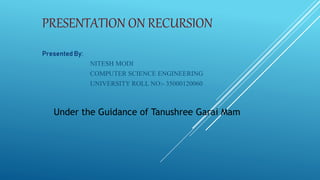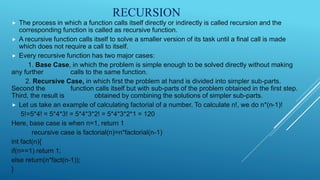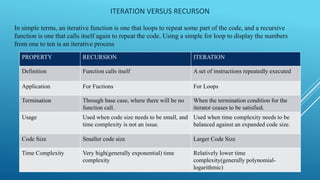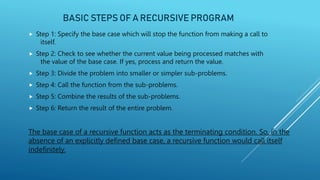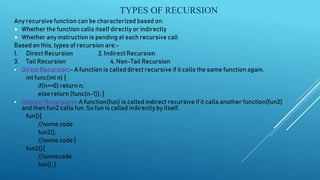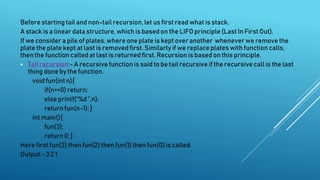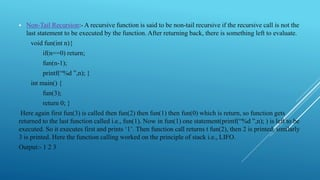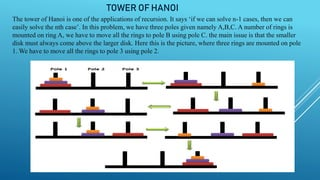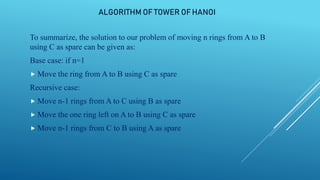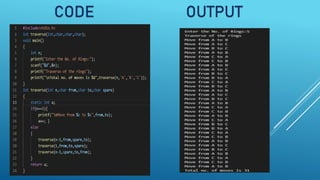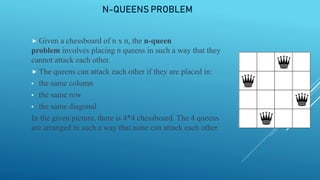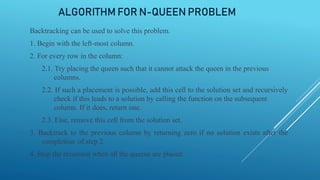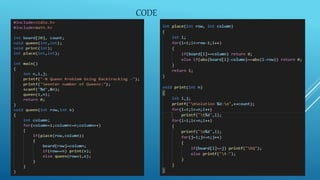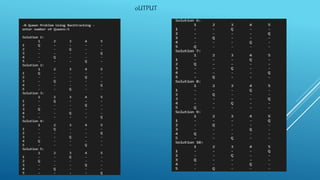1 de 15

### 35000120060_Nitesh Modi_CSE Presentation on recursion.pptx

• 1. PRESENTATION ON RECURSION Presented By: NITESH MODI COMPUTER SCIENCE ENGINEERING UNIVERSITY ROLL NO:- 35000120060 Under the Guidance of Tanushree Garai Mam
• 2. RECURSION  The process in which a function calls itself directly or indirectly is called recursion and the corresponding function is called as recursive function.  A recursive function calls itself to solve a smaller version of its task until a final call is made which does not require a call to itself.  Every recursive function has two major cases: 1. Base Case, in which the problem is simple enough to be solved directly without making any further calls to the same function. 2. Recursive Case, in which first the problem at hand is divided into simpler sub-parts. Second the function calls itself but with sub-parts of the problem obtained in the first step. Third, the result is obtained by combining the solutions of simpler sub-parts.  Let us take an example of calculating factorial of a number. To calculate n!, we do n*(n-1)! 5!=5*4! = 5*4*3! = 5*4*3*2! = 5*4*3*2*1 = 120 Here, base case is when n=1, return 1 recursive case is factorial(n)=n*factorial(n-1) int fact(n){ if(n==1) return 1; else return(n*fact(n-1)); }
• 3. ITERATION VERSUS RECURSON In simple terms, an iterative function is one that loops to repeat some part of the code, and a recursive function is one that calls itself again to repeat the code. Using a simple for loop to display the numbers from one to ten is an iterative process. PROPERTY RECURSION ITERATION Definition Function calls itself A set of instructions repeatedly executed Application For Fuctions For Loops Termination Through base case, where there will be no function call. When the termination condition for the iterator ceases to be satisfied. Usage Used when code size needs to be small, and time complexity is not an issue. Used when time complexity needs to be balanced against an expanded code size. Code Size Smaller code size Larger Code Size Time Complexity Very high(generally exponential) time complexity Relatively lower time complexity(generally polynomial- logarithmic)
• 4. BASIC STEPS OF A RECURSIVE PROGRAM  Step 1: Specify the base case which will stop the function from making a call to itself.  Step 2: Check to see whether the current value being processed matches with the value of the base case. If yes, process and return the value.  Step 3: Divide the problem into smaller or simpler sub-problems.  Step 4: Call the function from the sub-problems.  Step 5: Combine the results of the sub-problems.  Step 6: Return the result of the entire problem. The base case of a recursive function acts as the terminating condition. So, in the absence of an explicitly defined base case, a recursive function would call itself indefinitely.
• 5. TYPES OF RECURSION Any recursive function can be characterized based on:  Whether the function calls itself directly or indirectly  Whether any instruction is pending at each recursive call Based on this, types of recursion are:- 1. Direct Recursion 2. Indirect Recursion 3. Tail Recursion 4. Non-Tail Recursion  Direct Recursion:- A function is called direct recursive if it calls the same function again. int func(int n) { if(n==0) return n; else return (func(n-1)); }  Indirect Recursion:- A function(fun) is called indirect recursive if it calls another function(fun2) and then fun2 calls fun. So fun is called indirectly by itself. fun(){ //some code fun2(); //some code } fun2(){ //somecode fun(); }
• 6. Before starting tail and non-tail recursion, let us first read what is stack. A stack is a linear data structure, which is based on the LIFO principle (Last In First Out). If we consider a pile of plates, where one plate is kept over another wheneverwe remove the plate the plate kept at last is removed first. Similarly if we replace plates with function calls, then the function called at last is returned first. Recursion is based on this principle.  Tail recursion:- A recursive function is said to be tail recursive if the recursive call is the last thing done by the function. void fun(int n){ if(n==0) return; else printf(“%d ”,n); return fun(n-1); } int main(){ fun(3); return 0; } Here first fun(3) then fun(2) then fun(1) then fun(0) is called. Output:- 3 2 1
• 7.  Non-Tail Recursion:- A recursive function is said to be non-tail recursive if the recursive call is not the last statement to be executed by the function. After returning back, there is something left to evaluate. void fun(int n){ if(n==0) return; fun(n-1); printf(“%d ”,n); } int main() { fun(3); return 0; } Here again first fun(3) is called then fun(2) then fun(1) then fun(0) which is return, so function gets returned to the last function called i.e., fun(1). Now in fun(1) one statement(printf(“%d ”,n); ) is left to be executed. So it executes first and prints ‘1’. Then function call returns t fun(2), then 2 is printed, similarly 3 is printed. Here the function calling worked on the principle of stack i.e., LIFO. Output:- 1 2 3
• 8. TOWER OF HANOI The tower of Hanoi is one of the applications of recursion. It says ‘if we can solve n-1 cases, then we can easily solve the nth case’. In this problem, we have three poles given namely A,B,C. A number of rings is mounted on ring A, we have to move all the rings to pole B using pole C. the main issue is that the smaller disk must always come above the larger disk. Here this is the picture, where three rings are mounted on pole 1. We have to move all the rings to pole 3 using pole 2.
• 9. ALGORITHM OF TOWER OF HANOI To summarize, the solution to our problem of moving n rings from A to B using C as spare can be given as: Base case: if n=1  Move the ring from A to B using C as spare Recursive case:  Move n-1 rings from A to C using B as spare  Move the one ring left on A to B using C as spare  Move n-1 rings from C to B using A as spare
• 10. CODE OUTPUT
• 11. N-QUEENS PROBLEM  Given a chessboard of n x n, the n-queen problem involves placing n queens in such a way that they cannot attack each other.  The queens can attack each other if they are placed in: • the same column • the same row • the same diagonal In the given picture, there is 4*4 chessboard. The 4 queens are arranged in such a way that none can attack each other.
• 12. ALGORITHM FOR N-QUEEN PROBLEM Backtracking can be used to solve this​ problem. 1. Begin with the left-most column. 2. For every row in the column: 2.1. Try placing the queen such that it cannot attack the queen in the previous columns. 2.2. If such a placement is possible, add this cell to the solution set and recursively check if this leads to a solution by calling the function on the subsequent column. If it does, return one. 2.3. Else, remove this cell from the solution set. 3. Backtrack to the previous column by returning zero if no solution exists after the completion of step 2. 4. Stop the recursion when all the queens are placed.
• 13. CODE
• 14. 0UTPUT
• 15. THANK YOU